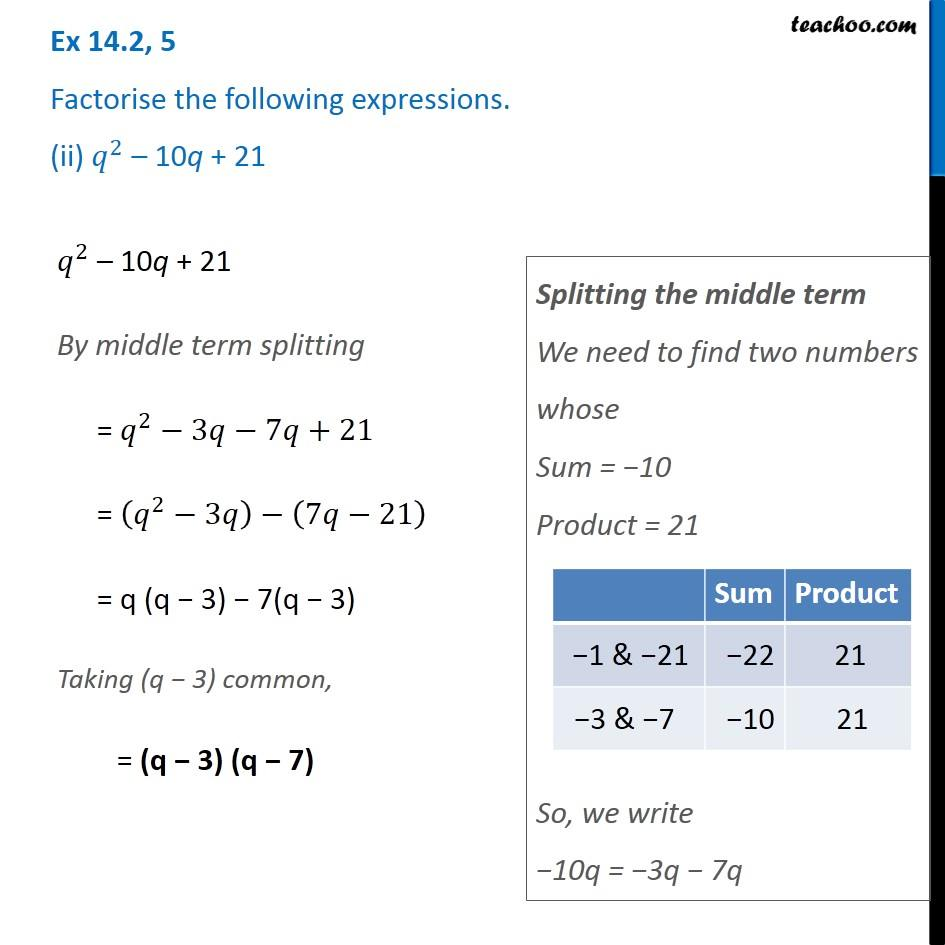Factorisation using splitting middle term

Chapter 14 Class 8 Factorisation
Concept wiseIntroducing your new favourite teacher - Teachoo Black, at only ₹83 per month

### Transcript

Ex 14.2, 5 Factorise the following expressions. (ii) 𝑞^2 – 10q + 21 𝑞^2 – 10q + 21 By middle term splitting = 𝑞^2−3𝑞−7𝑞+21 = (𝑞^2−3𝑞)−(7𝑞−21) = q (q − 3) − 7(q − 3) Taking (q − 3) common, = (q − 3) (q − 7) Splitting the middle term We need to find two numbers whose Sum = −10 Product = 21 So, we write −10q = −3q − 7q